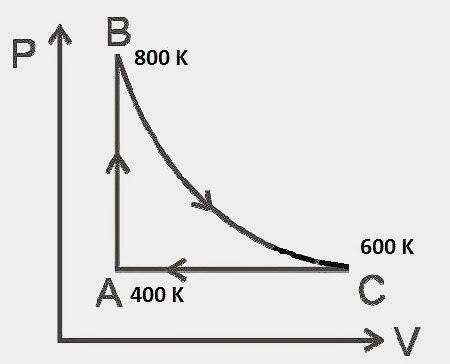## Tuesday, April 22, 2014

### JEE (Main) 2014 and JEE (Main) 2013 Multiple Choice Questions on Thermodynamics

"I object to violence because when it appears to do good, the good is only temporary; the evil it does is permanent."
– Mahatma GandhiToday we will discuss the multiple choice single answer type questions on thermodynamics, which appeared in JEE (Main) 2014 and JEE (Main) 2013 question papers.

(1) One mole of diatomic ideal gas undergoes a cyclic process ABC as shown in figure. The process BC is adiabatic. The temperatures at A, B and C are 400 K, 800 K and 600 K respectively. Choose the correct statement:

(1) The change in internal energy in the whole cyclic process is 250R

(2) The change in internal energy in the process CA is 700R

(3) The change in internal energy in the process AB is – 350R

(4) The change in internal energy in the process BC is – 500R
The change in the internal energy ΔU  is given by
ΔU =  n Cv ΔT where n is the number  of moles of gas, Cv is the molar specific heat of the gas at constant volume and ΔT is the change in the temperature of the gas.
The correct option is (4) since the change in internal energy in the process BC is 1×(5R/2) ×(–200) which is equal to – 500R.
[Note that the molar specific heat of diatomic ideal gas at constant volume is (5/2)R where R is the universal gas constant]
(2) The above P-V diagram represents the thermodynamic cycle of an engine, operating with an ideal monoatomic gas. The amount of heat extracted from the source in a single cycle is:
(1) P0V0
(2) (13/2) P0V0
(3) (11/2) P0V0
(4) 4 P0V0
The upward vertical portion (on the left of the P-V diagram) and the rightward horizontal portion (on the top of the P-V diagram) represent the extraction of heat by the gas from the source. In the first case, heat is extracted (from the source) at constant volume V0 (isochoric process) and in the second case, heat is extracted (from the source) at constant pressure 2P0 (isobaric process).
Heat absorbed (Q, let us say) from the source during the isochoric process is given by
Q = Cvn ΔT where Cv is the molar specific heat of the gas at constant volume, ΔT is the change in the temperature of the gas and n is the number of moles of gas in the engine.
Since PV = nRT where R is universal gas constant, we have
ΔT = (ΔP)V/nR = (2P0 P0)V0/nR
Heat absorbed from the source during the isochoric process is therefore given by
Q = Cvn (2P0 P0)V0/nR = (3/2)P0V0 since Cv = (3/2)R for an ideal monoatomic gas.
Heat absorbed (Q’, let us say) from the source during the isobaric process is given by
Q’ = Cpn ΔT where Cp is the molar specific heat of the gas at constant pressure.
Since PV = nRT and the varying quantities are V and T, we obtain
ΔT = P(ΔV)/nR = 2P0(2V0 – V0)/nR
Heat absorbed from the source during the isobaric process is therefore given by
Q’ = Cpn×2P0(2V0 – V0)/nR
Since  Cp = (5/2)R for an ideal monoatomic gas, we have
Q’ = 5P0V0
The total amount of heat extracted from the source in a single cycle is therefore given by
Q1 = Q + Q’ = (3/2)P0V0 + 5P0V0 = (13/2)P0V0
You can access all questions on thermodynamics posted on this site by clicking on the label ‘thermodynamics’ below this post.

Essential points to be remembered for working out questions in thermodynamics can be seen here.